# Binary LDM - Local Dissimilarity Map

Goal

For binary images, intensity information is poor and shape extraction is often difficult. Therefore binary images have to be compared without using feature extraction. The Local Dissimilarity Map (LDM) is an useful way to compare two binary images, providing a localized and quantified information. The LDM is computed from the following equation: $$\mathrm{LDM}_{A, B} (p) = \|A (p) - B (p) \| \max (\mathrm{dt}_A (p), \mathrm{dt}_B (p))$$ where $\mathrm{dt}_X$ is the distance transform of image $X$ (giving the distance to the nearest foreground pixel). For binary images, the equation can be simplified into : $$\mathrm{LDM}_{A, B} = B \mathrm{dt}_A + A \mathrm{dt}_B .$$

References

Examples

LDM(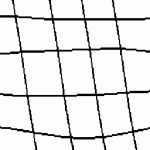,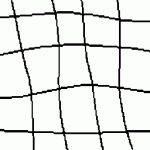)=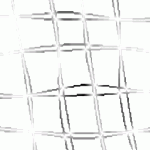(black is foreground)

LDM(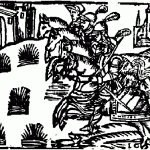,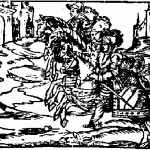)=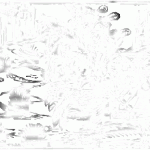(Images are from "L'histoire des Quatres fils Aymon" - see [Médiathèque de l'Agglomération Troyenne])

Java implementation
(Feel free to contact me for any question/remark/bug)

* Standalone jar file : LDMBinary.jar (sources included, see crestic/tip/LDM.java)
java -jar LDMBinary.jar ImageA ImageB [options]
(Please note that ImageA and ImageB are binarized if necessary)
Options usage:
-out LDM image (default: ldm.png)
-display Display images - no output
-help Describe command line

Sage notebook

Python code and test images : reference_implementation_ldm.sws.